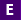#Not a Florida public school educator?

Access this resource
on CPALMS.com

### General Information

Subject(s): Mathematics, Science
Intended Audience: EducatorsSuggested Technology: Computer for Presenter, Computers for Students, Internet Connection, Adobe Acrobat Reader
Instructional Time: 40 Minute(s)
Keywords: slope, slope intercept, similar triangles, vertical line, non vertical line, proportional relationship, scratch program, scratch, slope is constant throughout the line, congruent triangles, program, coding, rise, run, rise over run, slope of vertical, slope of non-vertical line, scripting the program in scratch
Instructional Component Type(s):

## Aligned Standards

This vetted resource aligns to concepts or skills in these benchmarks.

## 2 Lesson Plans

Slope Intercept - Lesson #1

This is lesson 1 of 3 in the Slope Intercept unit. This lesson introduces graphing proportional relationships. In this lesson students will perform an experiment to find and relate density of two different materials to the constant of proportionality and unit rate.

Slope Intercept - Lesson #2

This is lesson 2 of 3 in the Slope Intercept unit. This lesson introduces graphing non-proportional linear relationships. In this lesson students will perform an activity to collect data to derive y = mx + b and will use a Scratch program to plot the graph of the data, as well as check for proportional and/or linear relationships.

## Related Resources

Other vetted resources related to this resource.

## Lesson Plans

Slope Intercept - Lesson #2:

This is lesson 2 of 3 in the Slope Intercept unit. This lesson introduces graphing non-proportional linear relationships. In this lesson students will perform an activity to collect data to derive y = mx + b and will use a Scratch program to plot the graph of the data, as well as check for proportional and/or linear relationships.

Type: Lesson Plan

Slope Intercept - Lesson #1:

This is lesson 1 of 3 in the Slope Intercept unit. This lesson introduces graphing proportional relationships. In this lesson students will perform an experiment to find and relate density of two different materials to the constant of proportionality and unit rate.

Type: Lesson Plan